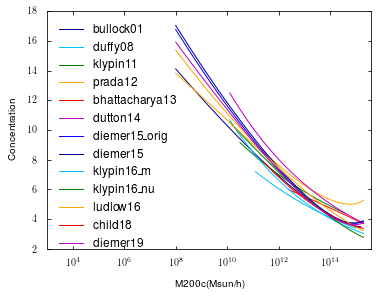# Colossus tutorial: halo concentration¶

Welcome to the Colossus concentration tutorial. Concentration is defined as the ratio of the outer radius of a halo to the scale radius, a concept that goes back to the Navarro, Frenk & White papers that proposed the NFW density profile. The Colossus concentration module implements a number of models for the mean or median concentration of halos.

In :
from __future__ import print_function
import numpy as np
import matplotlib.pyplot as plt
%matplotlib inline


As always with Colossus, we need to set a cosmology.

In :
from colossus.cosmology import cosmology
cosmology.setCosmology('planck15');


Let's import the concentration module:

In :
from colossus.halo import concentration


Now we can evaluate concentrations for a range of models of the c-M relation. First, let's list the models Colossus implements:

In :
for model_name in concentration.models:
print(model_name)

bullock01
duffy08
klypin11
bhattacharya13
dutton14
diemer15_orig
diemer15
klypin16_m
klypin16_nu
ludlow16
child18
diemer19


While there are functions for each of these models, the easier way to use the concentration module is through the unified concentration() interface. This function does a number of things: it converts between mass definitions, checks the validity of models and so on. Let's say we want to use the Bullock et al. 2001 model to get the concentration of a halo with virial mass $10^{12} M_{\odot}/h$ at $z = 0.5$:

In :
concentration.concentration(1E12, 'vir', 0.5, model = 'bullock01')

Out:
7.372645154916876

Of course, the function also accepts an array of masses, and returns an array of concentrations:

In :
M = 10**np.arange(10.0, 15.0, 1.0)
concentration.concentration(M, 'vir', 0.5, model = 'bullock01')

Out:
array([11.07869939,  9.14716812,  7.37264515,  5.79618839,  4.48465211])

However, not all models were constrained at all masses and redshifts, and Colossus can warn us in such cases:

In :
concentration.concentration(M, 'vir', 0.5, model = 'dutton14')

/Users/benedito/University/Codes/colossus_dev/colossus/halo/concentration.py:434: UserWarning: Some masses or redshifts are outside the validity of the concentration model.
warnings.warn('Some masses or redshifts are outside the validity of the concentration model.')

Out:
array([11.33628686,  9.32117855,  7.66427055,  6.30189013,  5.18168284])

The power-law model of Dutton & Maccio 2014 should probably not be used outside the range of masses where it was constrained. But which masses are valid and which aren't? Using the range_return parameter, we can get the function to return a mask that indicates the validity:

In :
c, mask = concentration.concentration(M, 'vir', 0.5, model = 'dutton14', range_return = True)

[False  True  True  True  True]
[9.32117855 7.66427055 6.30189013 5.18168284]


Only the lowest mass bin is outside the range, so we won't use that. One thing the concentration module does not check is the cosmology: if a model was calibrated only for a particular cosmology, it can still be evaluated even if a different cosmology is set. For example:

In :
concentration.concentration(1E12, 'vir', 0.5, model = 'duffy08')

Out:
6.226274912879475

The Duffy et al. 2008 model was calibrated for a WMAP5 cosmology, so care needs to be taken if we're working in a Planck 2015 cosmology (as set above). Let's put it all together and compare the models implemented in Colossus:

In :
cosmology.setCosmology('bolshoi')
M = 10**np.arange(8.0, 15.4, 0.1)

plt.figure()
plt.xscale('log')
plt.xlabel('M200c(Msun/h)')
plt.ylabel('Concentration')
for model_name in concentration.models:
c, mask = concentration.concentration(M, '200c', 0.0, model = model_name, range_return = True)

/opt/local/Library/Frameworks/Python.framework/Versions/3.5/lib/python3.5/site-packages/matplotlib/font_manager.py:1241: UserWarning: findfont: Font family ['sans-serif'] not found. Falling back to DejaVu Sans.